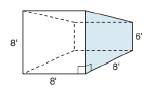Chapter 9.1, Problem 30E### Elementary Geometry for College St...

6th Edition
Daniel C. Alexander + 1 other
ISBN: 9781285195698

#### Solutions

Chapter
Section### Elementary Geometry for College St...

6th Edition
Daniel C. Alexander + 1 other
ISBN: 9781285195698
Textbook Problem
6 views

# A storage shed is in the shape of a trapezoidal prism. Each trapezoid represents one of its bases. With dimensions as shown, what is the storage capacity (volume) of its interior?To determine

To find:

The storage capacity (volume) of the interior of the storage shed in the shape of a trapezoidal prism.

Explanation

The volume of any solid is the region of space occupied by it and in general the volume of a solid is given by the product of its base area and altitude.

For a regular solid prism with base area B and altitude h, the volume is given by the formula

V=Bh.

Calculation:

Given,

The volume of the a prism with base area ‘B’ and height ‘h’ is given by the formula.

V=Bh

First let us calculate the base area of the prism.

The base of the prism is trapezoidal and its area is given by the formula.

B=12h(a+b)

Here, ‘a’ and ‘b’ are the length of the parallel sides of the trapezium and ‘h’ is the altitude of the trapezium.

From the given figure we have,

a=8 in

### Still sussing out bartleby?

Check out a sample textbook solution.

See a sample solution

#### The Solution to Your Study Problems

Bartleby provides explanations to thousands of textbook problems written by our experts, many with advanced degrees!

Get Started

#### Convert the expressions in Exercises 6584 to power form. 18xx23x35

Finite Mathematics and Applied Calculus (MindTap Course List)

#### Let g be the function defined by g(x)={12x+1ifx2x2ifx2 Find g(2), g(0), g(2), and g(4).

Applied Calculus for the Managerial, Life, and Social Sciences: A Brief Approach

#### Define the concept of external validity and a threat to external validity.

Research Methods for the Behavioral Sciences (MindTap Course List)

#### Sometime, Always, or Never:

Study Guide for Stewart's Single Variable Calculus: Early Transcendentals, 8th

#### Solve p20.1=1210.

Mathematics For Machine Technology